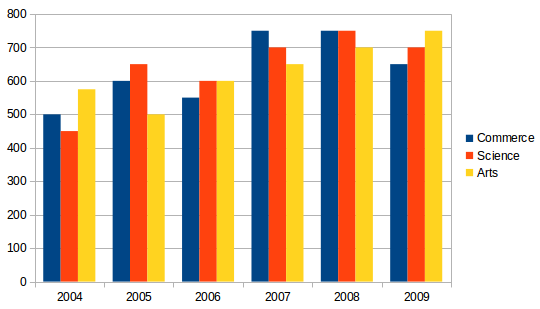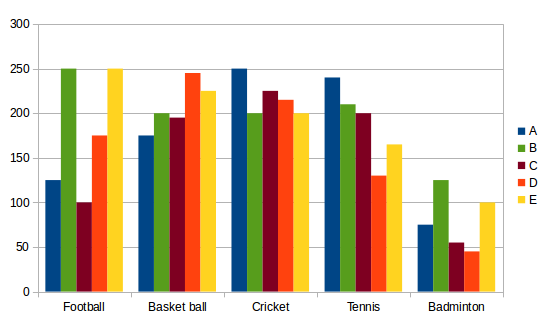## Data Interpretation For SBI PO : Set – 53

D.1-5) Study the following graph carefully and answer the questions:1) What is the ratio of the total number of students studying commerce in the years 2004 and 2006 together to that of those studying Arts in the year 2007 and 2009 together?

a) 3:4

b) 7:9

c) 4:3

d) 9:7

e) None of these

a)

The required ratio = (500+550)/(650+750)=1050/1400=3:4

2) What is the ratio of the total numbers of student studying Arts, Science and Commerce in all the years together?

a) 77:75:76

b) 76:75:77

c) 76:77:75

d) 75:77:76

e) None of these

d)

The required ratio = Arts : Science : Commerce

3750:3850:3800 = 75:77:76

3) The number of students studying Commerce in the year 2008 forms approximately what percent of the total number of students commerce in all the years together?

a) 13

b) 20

c) 29

d) 33

e) 38

e)

The required percent = (750/3800)*100=19.73%

4) What is the total number of students studying Arts in all the years together?

a) 3700

b) 2750

C) 3500

d) 2550

e) None of these

a)

The required number of students = 550+500+600+700+750=3750

5) The number of students studying  Science in the year 2009 forms what per cent of the total number of students studying all the disciplines together in that year?

a) 46.24

b) 23.51

c) 33.33

d) 32.56

e) None of these

c)

The required percent = (700)/(650+700+750)*100= (700/2100)*100=100/3=33.3%

D.6-10) Study the following table carefully and answer the questions6) If 20% of the Students playing Football from School ‘A’ also play Badminton, what should be the total number of students playing Badminton from School ‘A’?

a) 110

b) 120

c) 95

d) 100

e) None of these

d)

Total number of students playing in Badminton from school

A= 75+125*(20/100)=100

7) The number of Students playing Basketball from School ‘C’ is approximately what percent of the Students playing Basketball from School ‘E’?

a) 75

b) 87

c) 94

d) 70

e) 81

b)

The required percent =(195*100)/225= (195*4)/9= 86.67%

8) What is the difference between the average number of students playing Cricket from all the schools and the average number of students playing Tennis from all the schools?

a) 31

b) 26

c) 29

d) 33

e) None of these

c)

The required difference =(250-240)+(200-210)+(225-200)

(215-130)+(200-165)/5=145/5=29

9) The number of students playing Football from Schools ‘D’ is what percent of the total number of students playing all the given games that school?

a) 20.61

b) 21.59

c) 22.60

d) 20.59

e) None of these

e)

The required Percent ={175/(175+245+215+130+45)}*100= {175/810}*100= 21.60

10) What is the difference between the average number of students playing all the given games from school ‘B’ and the number of students playing Badminton from that school?

a) 72

b) 65

c) 78

d) 69

e) None of these